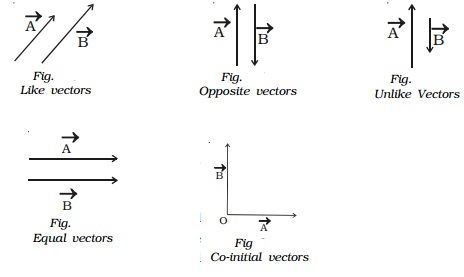Home | | Physics | Different types of vectors

# Different types of vectors(i) Equal vectors (ii) Like vectors (iii) Opposite vectors (iv) Unlike vectors (v) Unit vector and Orthogonal unit vectors (vi) Null vector or zero vector (vii) Proper vector (viii) Co-initial vectors (ix) Coplanar vectors

Different types of vectors

(i) Equal vectors

(ii) Like vectors

(iii) Opposite vectors

(iv) Unlike vectors

(v) Unit vector and Orthogonal unit vectors

(vi) Null vector or zero vector

(vii) Proper vector

(viii) Co-initial vectors

(ix) Coplanar vectors

(i) Equal vectors

Two vectors are said to be equal if they have the same magnitude and same direction, wherever be their

initial positions. In Fig.  the vectors �' A and �' B have the same magnitude and direction. Therefore �'  A and �' B are equal vectors.

(ii) Like vectors

Two vectors are said to be like vectors, if they have same direction but different magnitudes as shown in Fig. .

(iii) Opposite vectors

The vectors of same magnitude but opposite in direction, are called opposite vectors (Fig. ).

(iv) Unlike vectors

The vectors of different magnitude acting in opposite directions

are called unlike vectors. In Fig.  the vectors A and B are unlike vectors.(v) Unit vector

A vector having unit magnitude is called a unit vector. It is also defined as a vector divided by its own magnitude. A unit vector in the direction of a vector �' A,is written as Â and is read as 'A cap' or 'A caret' or 'A hat'. Therefore,Thus, a vector can be written as the product of its magnitude and unit vector along its direction.

Orthogonal unit vectors

There are three most common unit vectors in the positive directions of X,Y and Z axes of Cartesian coordinate system, denoted byi, j and respectively. Since they are along the mutually perpendicular directions, they are called orthogonal unit vectors.

(vi) Null vector or zero vector

A vector whose magnitude is zero, is called a null vector or zero vector. It is represented by 0 and its starting and end points are the same. The direction of null vector is not known.

(vii) Proper vector

All the non-zero vectors are called proper vectors.

(viii) Co-initial vectors

Vectors having the same starting point are called

co-initial vectors. In Fig. , �' A and �' B start from the same origin O. Hence, they are called as co-initial vectors.

(ix) Coplanar vectors

Vectors lying in the same plane are called coplanar vectors and the plane in which the vectors lie are called plane of vectors.

Study Material, Lecturing Notes, Assignment, Reference, Wiki description explanation, brief detail
11th 12th std standard Class Physics sciense Higher secondary school College Notes : Different types of vectors |